﻿ Parallel Lines
PARALLEL LINES
by Dr. Carol JVF Burns (website creator)
Follow along with the highlighted text while you listen!
• PRACTICE (online exercises and printable worksheets)

The blue and green lines below suggest parallel lines:

DEFINITION parallel lines
Two lines are parallel   if and only if   they lie in the same plane and do not intersect.

The condition ‘in the same plane’ is critical.
Two lines in three-dimensional space can certainly not intersect and yet not be parallel,
as the sides of the box below illustrate:

two lines (not in the same plane)
that don't intersect, but are not parallel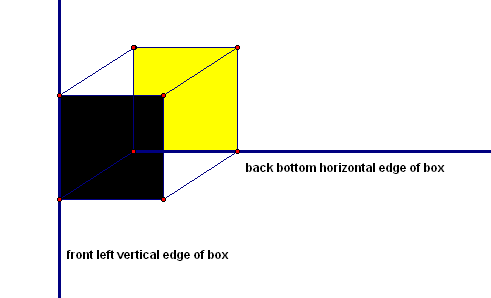Lines such as those illustrated above—that don't intersect, but are not parallel—are called skew lines:

DEFINITION skew lines
Two lines are skew   if and only if   they are not parallel and do not intersect.

This section explores conditions under which two lines are guaranteed to be parallel.

TRANSVERSALS AND ASSOCIATED ANGLES

First, we need an auxiliary line, called a transversal, that traverses or cuts across two coplanar lines: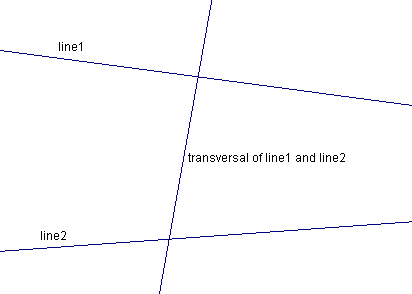This transversal brings lots of different angles into the picture, which are given names below.
Notice that the word alternate refers to being on opposite (alternate) sides of the transversal.
The word interior refers to being inside the two lines.
The word exterior refers to being outside the two lines.

ALTERNATE INTERIOR ANGLES are inside the lines, and on alternate sides of the transversal.
There are two pairs of alternate interior angles.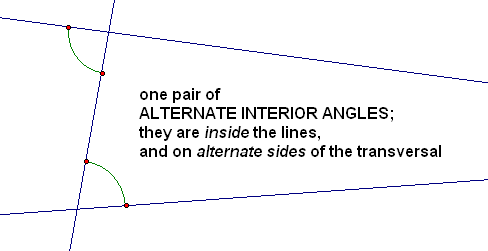ALTERNATE EXTERIOR ANGLES are outside the lines, and on alternate sides of the transversal.
There are two pairs of alternate exterior angles.SAME-SIDE INTERIOR ANGLES are inside the lines, and on the same side of the transversal.
There are two pairs of same-side interior angles.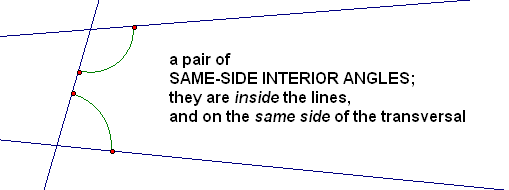CORRESPONDING ANGLES are in the same ‘quadrant’ formed by each line and the transversal.
In the sketch below, the ‘upper’ and ‘lower’ angles are both in ‘Quadrant I’.
There are four pairs of corresponding angles.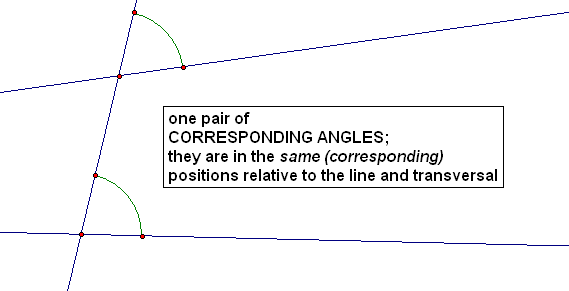When two lines are cut by a transversal, the angles named above can be used to decide if the lines are parallel or not, as follows:

THEOREM conditions for parallel lines
Suppose two lines are cut by a transversal.
Then, the following are equivalent:
• the two lines are parallel
• a pair of alternate interior angles are congruent
• a pair of alternate exterior angles are congruent
• a pair of corresponding angles are congruent
• a pair of same-side interior angles are supplementary

There is incredible power in a theorem of this sort!
Since these five statements are equivalent:

• if one of them is true, then all of them are true
• if one of them is false, then all of them are false

To prove that multiple statements are equivalent,
mathematicians often prove a chain of implications;
the chain starts and ends at the same statement, and covers all the statements in between.

For example, to prove that $\,A\Leftrightarrow B\text{ and }B\Leftrightarrow C\,$, one could prove any of the following:

• $\,A\Rightarrow B\,$ and $\,B\Rightarrow C\,$ and $\,C\Rightarrow A\,$
• $\,B\Rightarrow A\,$ and $\,A\Rightarrow C\,$ and $\,C\Rightarrow B\,$
• $\,C\Rightarrow B\,$ and $\,B\Rightarrow A\,$ and $\,A\Rightarrow C\,$

Here's a truth table which proves that $\,(A\Leftrightarrow B \text{ and } B\Leftrightarrow C)\,$ is equivalent to $\,(A\Rightarrow B\,$ and $\,B\Rightarrow C\,$ and $\,C\Rightarrow A)\,$.

(The mathematical word ‘and’ is associative, so we can say things like ‘$\,P\text{ and }Q\text{ and }R\,$’
Also, the only time that ‘$\,P\text{ and }Q\text{ and }R\,$’ is true is when all three subsentences are true.)

Since there are three individual statements ($\,A\,$, $\,B\,$, and $\,C\,$),
and two possible truth values for each (true or false),
there are $\,2^3 = 8\,$ rows in the truth table.

 $A$ $B$ $C$ $A\Leftrightarrow B$ $B\Leftrightarrow C$ $A\Leftrightarrow B\,$ and $\,B\Leftrightarrow C$ $A\Rightarrow B$ $B\Rightarrow C$ $C\Rightarrow A$ $A\Rightarrow B\,$ and $\,B\Rightarrow C$ and $\,C\Rightarrow A\,$ T T T T T T T T T T T T F T F F T F T F T F T F F F F T T F T F F F T F F T T F F T T F T F T T F F F T F F F F T F T F F F T T F F T T F F F F F T T T T T T T

Master the ideas from this section